GROUP THEORY SYMBOL

# GTBands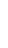GTBands[Hamiltionian,kpoints,nev]
calculates the lowest nev band energies for a given Hamiltonian at certain kpoints.

## DetailsDetails

• The Hamiltonian represents a matrix eigenvalue problem resulting from tight-binding or plane wave theory in electronic structure problems or as a result of the master equation in photonics. Also phonon can be considered.
• GTBands is used to decouple the calculation of the band structure from the plot. This is useful if large matrices have to be diagonalized as it is usually the case for photonic problems.
• The band structure can be calculated along paths in the Brillouin zone, i.e. data for a band structure plot are generated. On the other hand the eigenvalues on a mesh in the irreducible part of the Brillouin zone can be calculated as a first step to calculate the density of states.
• The following options can be given:
•  GODecimals 4 Controls the number of fractional digits in tables GOEigenvectors False Controls whether the eigenvectors will be calculated. GOPhotonic False Distinguishes between photonic and electronic bandstructure calculation. GOPlotBands True Controls calculation of energy bands in the Brillouin zone. GOShift 0 Shift the energies by a fixed value. GOStore 0 Controls output of bands and eigenvalues to files. GOTbOrthogonal True Controls if the calculation is a standard or general eigenvalue problem. GOVerbose False Controls the output of additional information.
• See

### Possible IssuesPossible Issues

for a small change in version 1.2.
• See: W. Hergert, M. Geilhufe, Group Theory in Solid State Physics and Photonics. Problem Solving with Mathematica, chapter 9.6.

## ExamplesExamplesopen allclose all

### Basic Examples  (1)Basic Examples  (1)

 In:=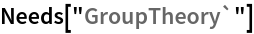The Hamiltonian will be prepared.

 In:=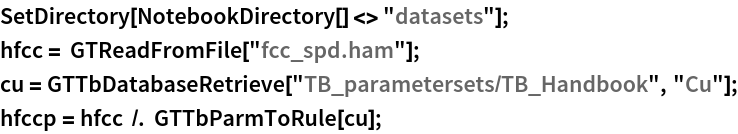A list of k-points will be prepared.

 In:=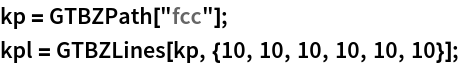The bands are calculated along the path.

 In:=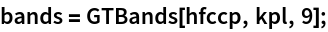This result can be used immediately to plot the band structure of Cu.

 In:=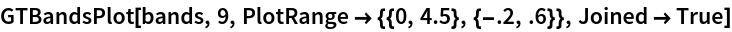Out=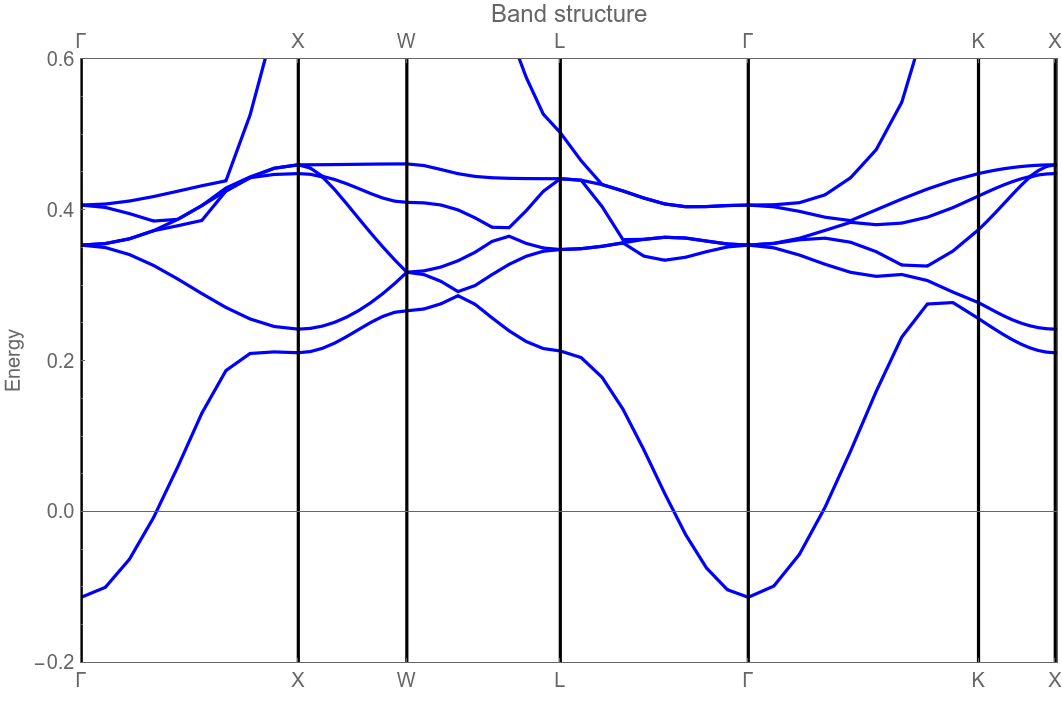### Possible Issues  (1)Possible Issues  (1)

GTBandsPlotGTBandStructureGTBZPathGTDensityOfStatesGTDensityOfStatesPlotGTPhFieldsGTPhMasterEquationGTTbHamiltonianGTFatBandsPlotGTPartialDOS